Legendre condition

A necessary condition for the solution of the simplest problem in variational calculus, proposed by A.M. Legendre in 1786: For the curveto provide a minimum of the functional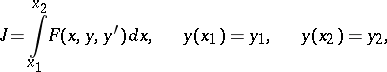it is necessary that at all points of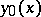the second derivative of the integrand with respect to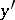should be non-negative: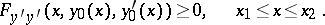Ifis an-dimensional vector with coordinates, then the Legendre condition requires that the quadratic form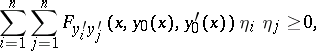should be non-negative. For the case of a maximum of the functional the sign of the inequality in the Legendre condition is reversed. For variational problems on a conditional extremum the analogue of the Legendre condition is the Clebsch condition.

The Legendre condition, like the Euler equation, is a necessary condition for a weak extremum. If the Legendre condition is violated, the second variation of the functional does not preserve its sign and the curvedoes not provide an extremum of the functional.

If in the Legendre condition the sign of non-strict inequality is replaced by the sign of strict inequality, then the condition is called the strong Legendre condition. The strong Legendre condition, in contrast to the Legendre condition, is not necessary. The strong Legendre condition is involved in the formulation of sufficient conditions for an extremum. An extremal on which the strong Legendre condition is satisfied is called a non-singular extremal. Such an extremal is twice continuously differentiable, and the Euler equation for it can be represented as an ordinary differential equation of the second order, solved for the highest derivative. If the strong Jacobi condition is satisfied on a non-singular extremal, then one can construct a field of extremals surrounding the given extremal, which is the first step in the investigation of sufficient conditions for an extremum.